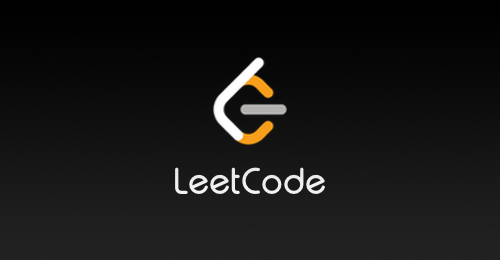# LeetCode 题解：15. 三数之和，JavaScript 双循环 +HashMap，详细注释Lee Chen#### 阅读更多系列文章请访问我的GitHub 博客

﻿

﻿

﻿

1. 第一层循环用于生成target，target=0-nums[i]，相当于两数之和中的target。

2. 第二层循环套用两数字和的一遍哈希表解法。

3. 该题需要去重，去重的要点是对数组进行排序，排序后的每个数字都已经归类。利用这个特点，可以直接排除掉大于0的数字，因为3个大于0的数字相加不可能等于0。

﻿

/** * @param {number[]} nums * @return {number[][]} */var threeSum = function (nums) {  let result = [];  // 先排序，防止顺序错误的失败  nums.sort((a, b) => a - b);  for (let i = 0; i < nums.length - 1; i++) {    // 当前值大于0时，3个数都大于0，3数之和必然大于0，无需判断    if (nums[i] > 0) {      break;    }    // 如果当前值与前一个值相等，即为已经处理过    // 而且由于是向前比较，因此不会出现遗漏，因为数组的遍历是向后的    if (nums[i - 1] === nums[i]) {      continue;    }    // target等同于tow-sum中的target    const target = 0 - nums[i];    let map = new Map();    for (let j = i + 1; j < nums.length; j++) {      // 与tow-sum不同，tow-sum不考虑可能出现相同解的情况，而该题需要考虑      // 由于nums[i]已经做过去重判断，此处只要判断结果的后两个值没有出现过即可      // 而由于nums已经排序过，只要对比上一个结果即可      if (result.length) {        if (          result[result.length - 1] === target - nums[j] &&          result[result.length - 1] === nums[j]        ) {          continue;        }      }      // tow-sum的判断方式，使用map进行判断      // 如果当前值已存在map中，则为当前结果。      if (typeof map.get(nums[j]) === 'number') {        result.push([nums[i], map.get(nums[j]), nums[j]]);      }      // 每次循环都缓存一次当前值，key为target - nums[j]      // 如果下次循环到的值与key相同，则可以直接取出之前的nums[j]      map.set(target - nums[j], nums[j]);    }  }  return result;};

﻿## 评论